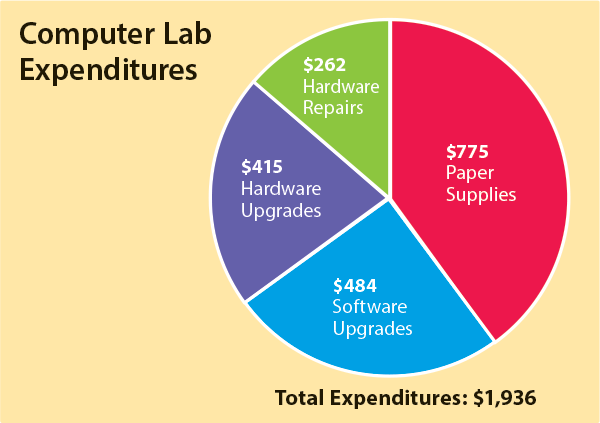Bookmark

# Creating Pie Graphs

## Creating Pie GraphsWhen you want to compare the parts of something to the whole, you can use a pie graph. This pie graph shows the amount of money that a school spent in one year on its computer lab. The total amount was \$1,936, and the greatest cost was paper supplies.

Your Turn Create a pie graph by hand.

1. Imagine that you have 30 students in your class and you poll them about their favorite pet type. Here are the results:
2.  Dog 10 Cat 6 Bird 5 Fish 3 Reptile 3 Rodent 3
3. Divide the number for each favorite pet type by the total number of students and multiply by 100 to get a percentage. Round anything after the decimal.
4. Multiply each favorite pet percentage by 3.6 to get the number of degrees.
5. Use a compass to draw a circle.
6. Draw a line from the top of the circle to the center.
7. Set a protractor on the line and measure to the right the number of degrees for the largest group.
8. Make a second line to mark the pie slice.
9. Set the protractor on this line and measure and mark the next largest pie slice.
10. Keep going from largest to smallest pie slice until you have completed the circle.
11. Label each pie slice with the pet type and percentage.
12. Give your pie graph a descriptive title.

1. Copy the data from the table above into a spreadsheet program, such as Google Sheets.
2. Find the spreadsheet function that lets you create a graph. (In Google Sheets, select the data, go to "Insert" and "Chart" and choose the pie graph.)

From pages 372-373 in Inquire Middle School

## Teacher Support:Answers Creating a Pie Graph.pdf